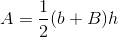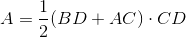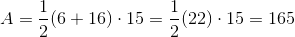PSAT Math : Trapezoids

Example Questions

Example Question #9 : How To Find The Area Of A Trapezoid

A trapezoid has a base of length 4, another base of length s, and a height of length s. A square has sides of length s. What is the value of s such that the area of the trapezoid and the area of the square are equal?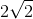Explanation:

In general, the formula for the area of a trapezoid is (1/2)(a + b)(h), where a and b are the lengths of the bases, and h is the length of the height. Thus, we can write the area for the trapezoid given in the problem as follows:

area of trapezoid = (1/2)(4 + s)(s)

Similarly, the area of a square with sides of length a is given by a2. Thus, the area of the square given in the problem is s2.

We now can set the area of the trapezoid equal to the area of the square and solve for s.

(1/2)(4 + s)(s) = s2

Multiply both sides by 2 to eliminate the 1/2.

(4 + s)(s) = 2s2

Distribute the s on the left.

4s + s2 = 2s2

Subtract s2 from both sides.

4s = s2

Because s must be a positive number, we can divide both sides by s.

4 = s

This means the value of s must be 4.

Example Question #1 : How To Find The Area Of A Trapezoid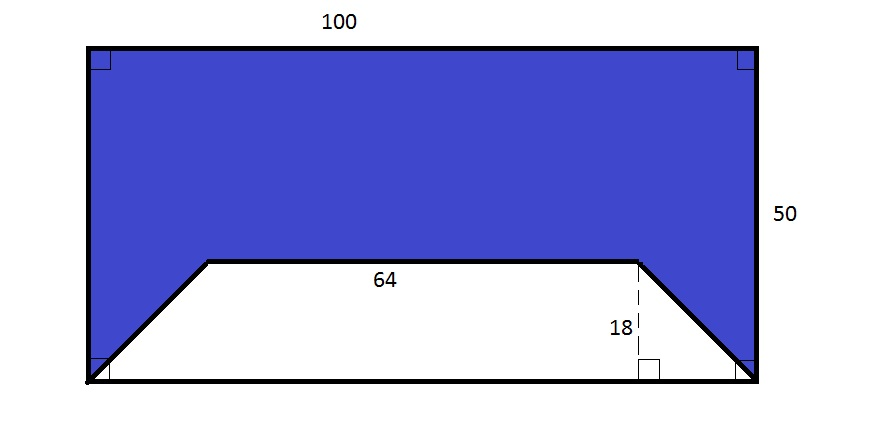Note: Figure NOT drawn to scale.

The white region in the above diagram is a trapezoid. What percent of the above rectangle, rounded to the nearest whole percent, is blue?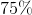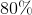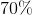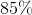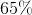Explanation:

The area of the entire rectangle is the product of its length and width, or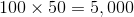.

The area of the white trapezoid is one half the product of its height and the sum of its base lengths, or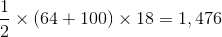Therefore, the blue polygon has area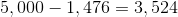This is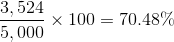of the rectangle.

Rounded, this is 70%.

Example Question #2 : How To Find The Area Of A Trapezoid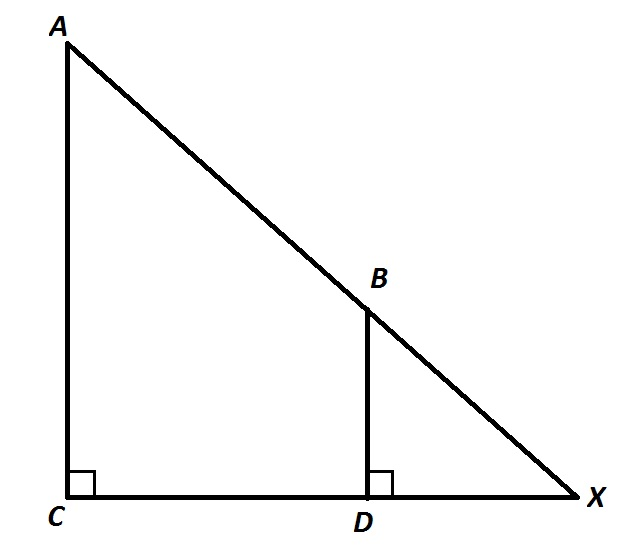Refer to the above diagram.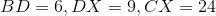.

Give the area of Quadrilateral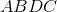.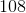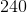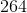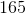Explanation: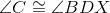, since both are right; by the Corresponding Angles Theorem,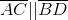, and Quadrilateralis a trapezoid.

By the Angle-Angle Similarity Postulate, sinceand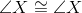(by reflexivity),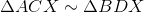and since corresponding sides of similar triangles are in proportion,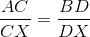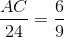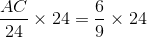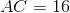, the larger base of the trapozoid;

The smaller base is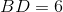.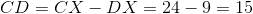, the height of the trapezoid.

The area of the trapezoid is